### Center of Gravity

The center of gravity is related to the center of mass.

All objects are subject to some kind of  torque at any given point in time about their center of mass due to their own force of gravity.

For all intensive purposes the center of mass will be the same as the center of gravity except when we analyze a body large enough such that the force of gravity is different in different parts of the object.
After all , if you consider that we are on a revolving object (the Earth) you will appreciate that all objects on this planet will have torque and  angular acceleration associated with them (with respect to some point in the universe) because of the force of gravity acting on them .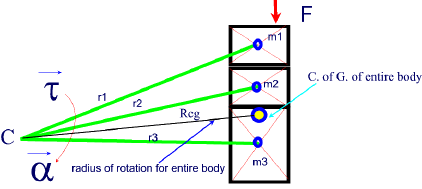Taking all the variables above into consideration, we can write: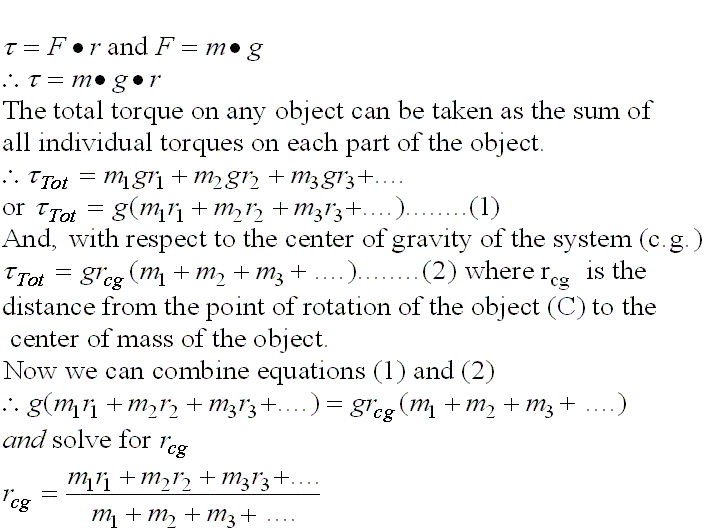Example1:

Calculate the center of gravity of the system shown below consisting of 5 objects with masses m1 to m5, 4.0 m apart from each other,  rotating about a fixed point C along a straght axis of rotation.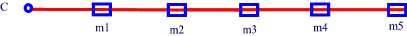Given:
m1= 20 Kg      m2= 10 Kg        m3 = 10 Kg       m4 = 40 Kg       m5 = 20 Kg
The masses are spaced 4.0 m apart.

Solution: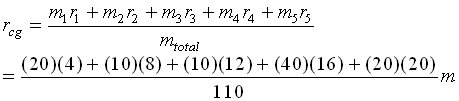Example2:

The center of gravity (cg) of a  1500 Kg  BMW is 3.00 m from the front seat.

Where will the cg be located when four passengers (60 Kg each) will occupy the vehicle.

Two passenger will seat in the front and two in the back.

The front seats are 2.0 m from the  front of the car and the back seats are 3.6 m from the front of the car.

Given:

m1 = 1500 Kg        m2 = 2X 60 =120 Kg         m3 = 2 X 60 = 120 Kg
r1 = 3.00 m              r2 = 2.0 m                            r3 = 3.6 m

Find: rcg

Solution: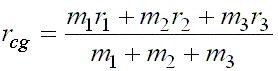= 2.97 m